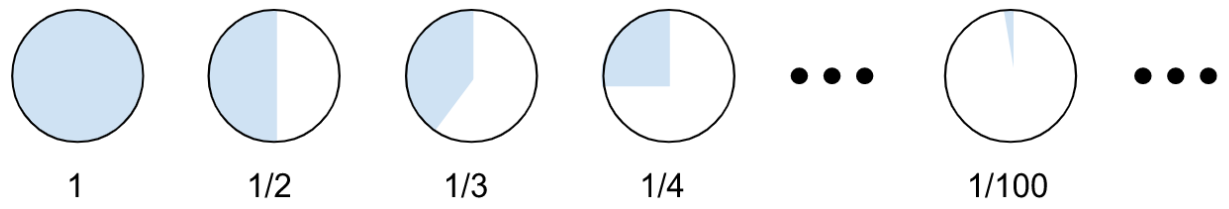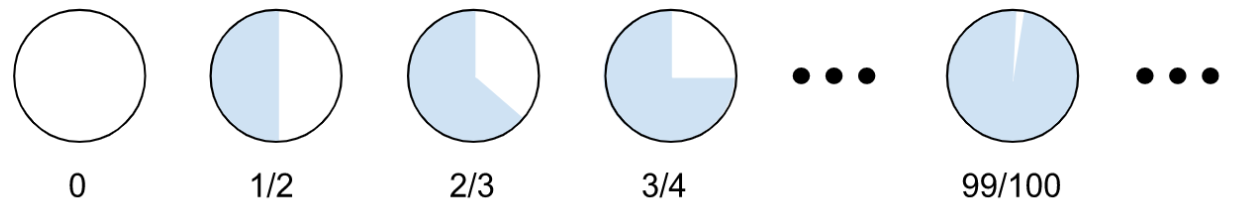# Intuiting Sequences

This post is part of a series.

A sequence is a list of numbers that has some pattern. For example, $2, 4, 6, 8, \ldots$ is the sequence of even numbers, and $1, 3, 5, 7, \ldots$ is the sequence of odd numbers.

We can interpret sequences as functions evaluated at whole numbers. For example, the sequence of even numbers is like a function $y=2x,$ where $x$ is evaluated at $1, 2, 3, 4, \ldots.$ The result is $2(1), 2(2), 2(3), 2(4), \ldots$ which simplifies to $2, 4, 6, 8, \ldots.$

Because we’re only plugging in whole numbers for $x,$ we usually use the term $n$ instead of $x,$ and $a_n$ instead of $y.$ So the sequence of even numbers is given by the rule $a_n = 2n,$ as follows:

\begin{align*} a_1 &= 2(1) = 2 \\[5pt] a_2 &= 2(2) = 4 \\[5pt] a_3 &= 2(3) = 6 \\[5pt] a_4 &= 2(4) = 8 \\[5pt] \vdots \end{align*}

To check your understanding, observe that the sequence of odd numbers is given by $a_n = 2n-1{:}$

\begin{align*} a_1 &= 2(1) - 1 = 2 - 1 = 1 \\[5pt] a_2 &= 2(2) - 1 = 4 - 1 = 3 \\[5pt] a_3 &= 2(3) - 1 = 6 - 1 = 5 \\[5pt] a_4 &= 2(4) - 1 = 8 - 1 = 7 \\[5pt] \vdots \end{align*}

The sequences of odd and even numbers keep getting bigger as they go on, but not all sequences are like that. For example, consider the sequence $a_n = \frac{1}{n}.$ This sequence, which starts off $1, \frac{1}{2}, \frac{1}{3}, \frac{1}{4}, \ldots$ is a sequence of fractions that keeps on getting smaller.

Not only does this sequence get smaller, but it also gets closer and closer to a single number as the sequence goes on. That number is zero – we say that the sequence converges to zero.Likewise, consider the sequence $a_n=\frac{n-1}{n}.$ This sequence is an increasing sequence that gets closer and closer to $1,$ so we say it converges to $1.$You can usually tell whether a sequence converges by thinking about what happens when you plug in very large numbers for $n.$ For example, the sequence $a_n=\frac{1}{n}$ converges to $0,$ and plugging in a million for $n$ gives

\begin{align*} a_{1000000}=\frac{1}{1000000}=0.000001, \end{align*}

which is really close to $0.$ Likewise, the sequence $a_n=\frac{n-1}{n}$ converges to $1,$ and plugging in a million for $n$ gives

\begin{align*} a_{1000000}=\frac{99999}{1000000}=0.99999, \end{align*}

which is really close to $1.$

This post is part of a series.

Tags: